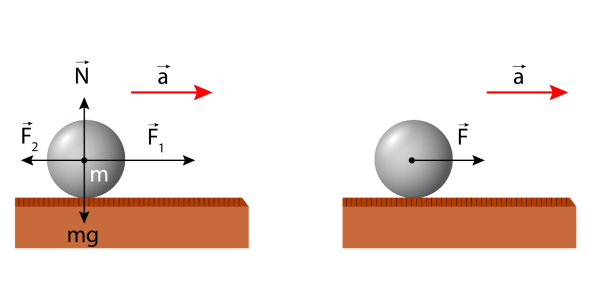# JEE Main Physics Quiz: Laws Of Motion Set 2!

10 Questions | Attempts: 111
ShareSettings.

• 1.
A block of mass 0.2 kg is held against a wall by applying a horizontal force of 6 N on the block. If the coefficient of friction between the block and the wall is 0.3, then what will be the value of frictional force acting on the block?
• A.

1.8 N

• B.

0.49 N

• C.

2.45 N

• D.

1.96 N

• 2.
During the motion of the bicycle, a force of friction is exerted by the ground on the two wheels of the bicycle. Which of the following statement is true in this context?
• A.

Frictional force for the wheels of bicycle will depend on the motion of the wheels

• B.

Frictional force will depend on the surface and the weight of bicycle and the person sitting on it

• C.

Frictional force will act in the direction of the motion

• D.

None of these

• 3.
An ant is slowly crawling up a hemispherical surface with negligible speed. The coefficient of friction between the ant and the surface is 2/3. If the line joining the ant and the center of the hemispherical surface subtends an angle ‘θ’ with vertical, then which of the following is true?
• A.

Cot θ = 1.5

• B.

Tan θ = 1.5

• C.

Sec θ = 1.5

• D.

Cosec θ = 1.5

• 4.
A block is thrown on the arrangement shown, with some initial velocity. The coefficient of friction between the conveyer belt and the box is μ. With what velocity the box should be projected initially so that it just reaches to end B.
• A.
• B.
• C.
• D.
• 5.
In the diagram shown the shaded block is fixed. The mass of block A is 4 kg and the coefficient of friction between the shaded block and block A is 1/4. What should be the maximum weight of the block b so that the whole system remains in the state of equilibrium?
• A.

10 N

• B.

40 N

• C.

20 N

• D.

15 N

• 6.
In the diagram weight of A = 250 N, the weight of B = 150 N, the weight of C = 100 N. The value of the coefficient of friction between A & B is 0.7 and that is between B & C is 0.4 and between C and the ground, the coefficient of friction is 0.3. What should be the minimum value of force F so that any part of the system shown in the figure starts moving?
• A.

175 N

• B.

90 N

• C.

160 N

• D.

150 N

• 7.
A particle starts from point C with some initial velocity in the hemispherical vessel of radius ‘R’. Mass of the particle is ‘m’ and the coefficient of friction between the particle and the hemispherical surface is μ then what should be the value of initial velocity so that the particle starts falling after reaching the point B.
• A.
• B.
• C.
• D.
• 8.
A passenger is traveling in a train moving at a speed of 50 m/s. Briefcase of the man is resting on the berth of the train. Suddenly the driver applies brakes due to which the speed of the train decreases at a speed of 15 m/s in 3 s. What should be the minimum coefficient of friction between the berth and the briefcase so that the briefcase doesn’t slide down? (take g = 10 m/s2)
• A.

0.1

• B.

0.5

• C.

0.2

• D.

2

• 9.
Which of the following statement is wrong about the friction?
• A.

The formula f = μN tells us that the f and N are comparable as they are having these two quantities have the same direction

• B.

Friction force can be zero, even though the surfaces in contact are rough

• C.

If there is no relative motion between the two surfaces still there might be a friction force between the surfaces

• D.

None of these

• 10.
Two blocks A and B of different masses have velocities VA and VB respectively. A horizontal force F is acting on the block A. Coefficient of friction between A & B is μ and that of between B & ground is zero. If VA = VB then which among the following statement is correct?
• A.

Friction on A supports its motion

• B.

Friction on B supports its motion

• C.

Both a & b

• D.

None of these

## Related TopicsBack to top
×

Wait!
Here's an interesting quiz for you.